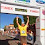## Friday, November 13, 2009

### Draw Three

Below there are sixteen numbers. Assuming that any three of the numbers may be drawn at random, what are the chances that three numbers will be drawn whose sum equals six?

1 2 3 1
2 3 1 2
3 1 2 3
1 2 3 1

1.As previously stated, I'm no stats genius, but here's my guess. Probability says:

The probability of any one of several distinct events is the sum of their individual probabilities, provided that the events are mutually exclusive (i.e. occurrence of one event precludes the others, e.g. selection without replacement).

So I would say that the odds of drawing three tiles that total 6 are the sum of their individual probabilities.

Out of the 27 possible combinations of drawing three tiles, we can get 6 by drawing:
1. 1-2-3
2. 1-3-2
3. 2-1-3
4. 2-2-2
5. 2-3-1
6. 3-1-2
7. 3-2-1

I found the probability of drawing each one of those is:
1. .044643 (6/16*5/15*5/14) and so on for each
2. .044643
3. .044643
4. .017857
5. .044643
6. .044643
7. .044643

The sum of all those probabilities is .285714.

So that's my guess, about 29% chance of drawing three that add up to 6.

2.Same answer as the pilot, but I got there a different way.

Using the combination formula for choosing from a set where order doesn't matter, nCr=

n!
--------
r!(n-r)!

Total combinations = 16C3 = 560

I wasn't sure how to figure out the right combinations, so I subtracted the ones that aren't right:

All three 1's = 6C3 = 20
All three 3's = 5C3 = 10
Two 1's and a 2 or 3 = 6C2 * 10 = 150
Two 2's and a 1 or 3 = 5C2 * 11 = 110
Two 3's and a 1 or 2 = 5C2 * 11 = 110

560-20-10-150-110-110 = 160

So the odds of drawing a total value of 6 is:

160/560 = .285714 = about 29%

3.To get 6 we either need '123' (in any order) or '222'.

P(123 in any order) = 6*P(123 in that order) = 6*(6*5*5)/(16*15*14) = 15/56
P(222) = (5*4*3)/(16*15*14) = 1/56

so P(sum is a 6) = 16/56 = 2/7
which is, as calculated in the previous posts, around 0.2857.

4.I love the variation on how you solved the answers. I can't add anything more to what you've written, but the answer is 0.2857 or so.

I love coming back from a weekend like this one to find this type of answer.

Leave your answer or, if you want to post a question of your own, send me an e-mail. Look in the about section to find my e-mail address. If it's new, I'll post it soon.

Please don't leave spam or 'Awesome blog, come visit mine' messages. I'll delete them soon after.

Enter your Email and join hundreds of others who get their Question of the Day sent right to their mailbox

The Lamplight Manor Puzz 3-D